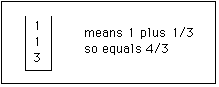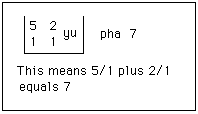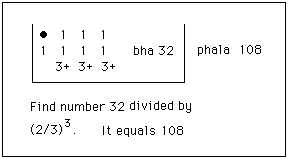# The Bakhshali manuscript

The Bakhshali manuscript is an early mathematical manuscript which was discovered over 100 years ago. We shall discuss in a moment the problem of dating this manuscript, a topic which has aroused much controversy, but for the moment we will examine how it was discovered. The paper  describes this discovery along with the early history of the manuscript. Gupta writes:-
The Bakhshali Manuscript is the name given to the mathematical work written on birch bark and found in the summer of 1881 near the village Bakhshali (or Bakhshalai) of the Yusufzai subdivision of the Peshawar district (now in Pakistan). The village is in Mardan tahsil and is situated 50 miles from the city of Peshawar.

An Inspector of Police named Mian An-Wan-Udin (whose tenant actually discovered the manuscript while digging a stone enclosure in a ruined place) took the work to the Assistant Commissioner at Mardan who intended to forward the manuscript to Lahore Museum. However, it was subsequently sent to the Lieutenant Governor of Punjab who, on the advice of General A Cunningham, directed it to be passed on to Dr Rudolf Hoernle of the Calcutta Madrasa for study and publication. Dr Hoernle presented a description of the BM before the Asiatic Society of Bengal in 1882, and this was published in the Indian Antiquary in 1883. He gave a fuller account at the Seventh Oriental Conference held at Vienna in 1886 and this was published in its Proceedings. A revised version of this paper appeared in the Indian Antiquary of 1888. In 1902, he presented the Bakhshali Manuscript to the Bodleian Library, Oxford, where it is still (Shelf mark: MS. Sansk. d. 14).
A large part of the manuscript had been destroyed and only about 70 leaves of birch-bark, of which a few were only scraps, survived to the time of its discovery.

To show the arguments regarding its age we note that F R Hoernle, referred to in the quotation above, placed the Bakhshali manuscript between the third and fourth centuries AD. Many other historians of mathematics such as Moritz Cantor, F Cajori, B Datta, S N Sen, A K Bag, and R C Gupta agreed with this dating. In 1927-1933 the Bakhshali manuscript was edited by G R Kaye and published with a comprehensive introduction, an English translation, and a transliteration together with facsimiles of the text. Kaye claimed that the manuscript dated from the twelfth century AD and he even doubted that it was of Indian origin.

Channabasappa in  gives the range 200 - 400 AD as the most probable date. In  the same author identifies five specific mathematical terms which do not occur in the works of Aryabhata and he argues that this strongly supports a date for the Bakhshali manuscript earlier than the 5th century. Joseph in  suggests that the evidence all points to the:-
... manuscript [being] probably a later copy of a document composed at some time in the first few centuries of the Christian era.
L V Gurjar in  claims that the manuscript is no later than 300 AD. On the other hand T Hayashi claims in  that the date of the original is probably from the seventh century, but he also claims that the manuscript itself is a later copy which was made between the eighth and the twelfth centuries AD.

I [EFR] feel that if one weighs all the evidence of these experts the most likely conclusion is that the manuscript is a later copy of a work first composed around 400 AD. Why do I believe that the actual manuscript was written later? Well our current understanding of Indian numerals and writing would date the numerals used in the manuscript as not having appeared before the ninth or tenth century. To accept that this style of numeral existed in 400 AD. would force us to change greatly our whole concept of the time-scale for the development of Indian numerals. Sometimes, of course, we are forced into major rethinks but, without supporting evidence, everything points to the manuscript being a tenth century copy of an original from around 400 AD. Despite the claims of Kaye, it is essentially certain that the manuscript is Indian.

The attraction of the date of 400 AD for the Bakhshali manuscript is that this puts it just before the "classical period" of Indian mathematics which began with the work of Aryabhata I around 500. It would then fill in knowledge we have of Indian mathematics for, prior to the discovery of this manuscript, we had little knowledge of Indian mathematics between the dates of about 200 BC. and 500 AD. This date would make it a document near the end of the period of Jaina mathematics and it can be seen as, in some sense, marking the achievements of the Jains.

What does the manuscript contain? Joseph writes in :-
The Bakhshali manuscript is a handbook of rules and illustrative examples together with their solutions. It is devoted mainly to arithmetic and algebra, with just a few problems on geometry and mensuration. Only parts of it have been restored, so we cannot be certain about the balance between different topics.
Now the way that the manuscript is laid out is quite unusual for an Indian document (which of course leads people like Kaye to prefer the hypothesis that it is not Indian at all - an idea in which we cannot see any merit). The Bakhshali manuscript gives the statement of a rule. There then follows an example given first in words, then using mathematical notation. The solution to the example is then given and finally a proof is set out.

The notation used is not unlike that used by Aryabhata but it does have features not found in any other document. Fractions are not dissimilar in notation to that used today, written with one number below the other. No line appears between the numbers as we would write today, however. Another unusual feature is the sign + placed after a number to indicate a negative. It is very strange for us today to see our addition symbol being used for subtraction. As an example, here is how $\large\frac{3}{4}\normalsize - \large\frac{1}{2}\normalsize$ would be written.3 /4 minus 1 /2

Compound fractions were written in three lines. Hence 1 plus 1 /3 would be written thus1 plus 1 /3

and 1 minus 1 /3 = 2 /3 in the following way1 minus 1 /3 = 2 /3

Sums of fractions such as 5 /1 plus 2 /1 are written using the symbol yu ( for yuta)5 /1 plus 2 /1

Division is denoted by bha, an abbreviation for bhaga meaning "part". For example8 divided by 2 /3

Equations are given with a large dot representing the unknown. A confusing aspect of Indian mathematics is that this notation was also often used to denote zero, and sometimes this same notation for both zero and the unknown are used in the same document. Here is an example of an equation as it appears in the Bakhshali manuscript.Equation

The method of equalisation is found in many types of problems which occur in the manuscript. Problems of this type which are found in the manuscript are examined in  and some of these lead to indeterminate equations. Included are problems concerning equalising wealth, the positions of two travellers, wages, and purchases by a number of merchants. These problems can all be reduced to solving a linear equation with one unknown or to a system of n linear equations in n unknowns. To illustrate we give the following indeterminate problem which, of course, does not have a unique solution:-
One person possesses seven asava horses, another nine haya horses, and another ten camels. Each gives two animals, one to each of the others. They are then equally well off. Find the price of each animal and the total value of the animals possesses by each person.
The solution, translated into modern notation, proceeds as follows. We seek integer solutions $x_{1}, x_{2}, x_{3}$ and $k$ (where $x_{1}$ is the price of an asava, $x_{2}$ is the price of a haya, and $x_{3}$ is the price of a horse) satisfying
$5 x_{1} + x_{2} + x_{3} = x_{1} + 7 x_{2}+ x_{3} = x_{1} + x_{2} + 8 x_{3} = k$.
Then $4 x_{1} = 6 x_{2} = 7 x_{3} = k - (x_{1} + x_{2} + x_{3})$.

For integer solutions $k - (x_{1} + x_{2} + x_{3})$ must be a multiple of the lcm of 4, 6 and 7. This is the indeterminate nature of the problem and taking different multiples of the lcm will lead to different solutions. The Bakhshali manuscript takes $k - (x_{1} + x_{2} + x_{3}) = 168$ (this is 4 × 6 × 7) giving $x_{1} = 42, x_{2} = 28, x_{3} = 24$. Then $k = 262$ is the total value of the animals possesses by each person. This is not the minimum integer solution which would be $k = 131$.

If we use modern methods we would solve the system of three equation for $x_{1}, x_{2}, x_{3}$ in terms of $k$ to obtain
$x_{1} = 21k/131, x_{2} = 14k/131, x_{3} = 12k/131$
so we obtain integer solutions by taking $k = 131$ which is the smallest solution. This solution is not given in the Bakhshali manuscript but the author of the manuscript would have obtained this had he taken $k - (x_{1} + x_{2} + x_{3})$ = lcm(4, 6, 7) = 84.

Here is another equalisation problem taken from the manuscript which has a unique solution:-
Two page-boys are attendants of a king. For their services one gets $\large\frac{13}{6}\normalsize$ dinaras a day and the other $\large\frac{3}{2}\normalsize$. The first owes the second 10 dinaras. calculate and tell me when they have equal amounts.
Now I would solve this by saying that the first gets $\large\frac{13}{6}\normalsize - \large\frac{3}{2}\normalsize = \large\frac{2}{3}\normalsize$ dinaras more than the second each day. He needs 20 dinaras more than the second to be able to give back his 10 dinaras debt and have them with equal amounts. So 30 days are required when each has $13 \times \large\frac{30}{6}\normalsize - 10 = 55$ dinaras. This is not the method of the Bakhshali manuscript which uses the "rule of three".

The rule of three is the familiar way of solving problems of the type: if a man earns 50 dinaras in 8 days how much will he earn in 12 days. The Bakhshali manuscript describes the rule where the three numbers are written
8 50 12
The 8 is the "pramana", the 50 is the "phala" and the 12 is the "iccha". The rule, according to the Bakhshali manuscript gives the answer as
phala × iccha/pramana
or in the case of the example $50 \times \large\frac{12}{8}\normalsize = 75$ dinaras.

Applying this to the page-boy problem we obtain equal amounts for the page-boys after $n$ days where
$13 \times \large\frac{n}{6}\normalsize = 3 \times \large\frac{n}{2}\normalsize +20$
so $n = 30$ and each has $13 \times \large\frac{30}{6}\normalsize - 10 = 55$ dinaras.

Another interesting piece of mathematics in the manuscript concerns calculating square roots. The following formula is used
$√Q = √(A^{2} + b) = A + b/2A - (b/2A)^{2}/(2(A + b/2A))$
This is stated in the manuscript as follows:-
In the case of a non-square number, subtract the nearest square number, divide the remainder by twice this nearest square; half the square of this is divided by the sum of the approximate root and the fraction. this is subtracted and will give the corrected root.
Taking $Q = 41$, then $A = 6, b = 5$ and we obtain 6.403138528 as the approximation to √41 = 6.403124237. Hence we see that the Bakhshali formula gives the result correct to four decimal places.

The Bakhshali manuscript also uses the formula to compute √105 giving 10.24695122 as the approximation to √105 = 10.24695077. This time the Bakhshali formula gives the result correct to five decimal places.

The following examples also occur in the Bakhshali manuscript where the author applies the formula to obtain approximate square roots:
487
Bakhshali formula gives 22.068076490965
Here 9 decimal places are correct

889
Bakhshali formula gives 29.816105242176
Here 5 decimal places are correct

[Note. If we took $889 = 30^{2} - 11$ instead of $29^{2} + 48$ we would get
Bakhshali formula gives 29.816103037078
Here 8 decimal places are correct]

339009
Bakhshali formula gives 582.2447938796899
Here 11 decimal places are correct
It is interesting to note that Channabasappa  derives from the Bakhshali square root formula an iterative scheme for approximating square roots. He finds in  that it is 38% faster than Newton's method in giving √41 to ten places of decimals.

### References (show)

1. L V Gurjar, Ancient Indian Mathematics and Vedha (Poona, 1947).
2. T Hayashi, The Bakhshali manuscript : An ancient Indian mathematical treatise (Groningen, 1995).
3. G G Joseph, The crest of the peacock (London, 1991).
4. C N Srinivasiengar, The history of ancient Indian mathematics (Calcutta, 1967).
5. M N Channabasappa, Mathematical terminology peculiar to the Bakhshali manuscript, Ganita Bharati 6 (1-4) (1984), 13-18.
6. M N Channabasappa, On the square root formula in the Bakhshali manuscript, Indian J. History Sci. 11 (2) (1976), 112-124.
7. M N Channabasappa, The Bakhshali square-root formula and high speed computation, Ganita Bharati 1 (3-4) (1979), 25-27.
8. R C Gupta, Centenary of Bakhshali manuscript's discovery, Ganita Bharati 3 (3-4) (1981), 103-105.
9. R C Gupta, Some equalization problems from the Bakhshali manuscript, Indian J. Hist. Sci. 21 (1) (1986), 51-61.
10. R Sarkar, The Bakhshali manuscript, Ganita Bharati 4 (1-2) (1982), 50-55.

Written by J J O'Connor and E F Robertson
Last Update November 2000